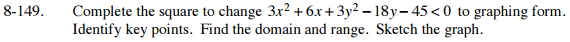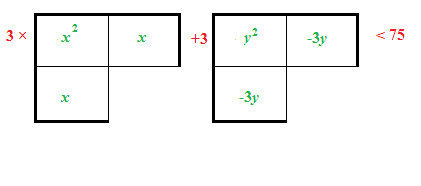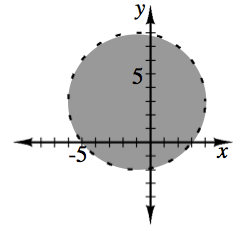### Home > A2C > Chapter 8 > Lesson 8.2.3 > Problem8-149

8-149.

Complete the square to change 3x2 + 6x + 3y2 − 18y − 45 < 0 to graphing form. Identify key points. Find the domain and range. Sketch the graph. Homework Help ✎3(x2 + 2x) + 3(y2 − 6y) − 45 < 0

3(x + 1)2 + 3(y − 3)2 − 75 < 0

Divide by 3, then simplify.(x + 1)2 + (y − 3)2 < 25
Center = (−1, 3)